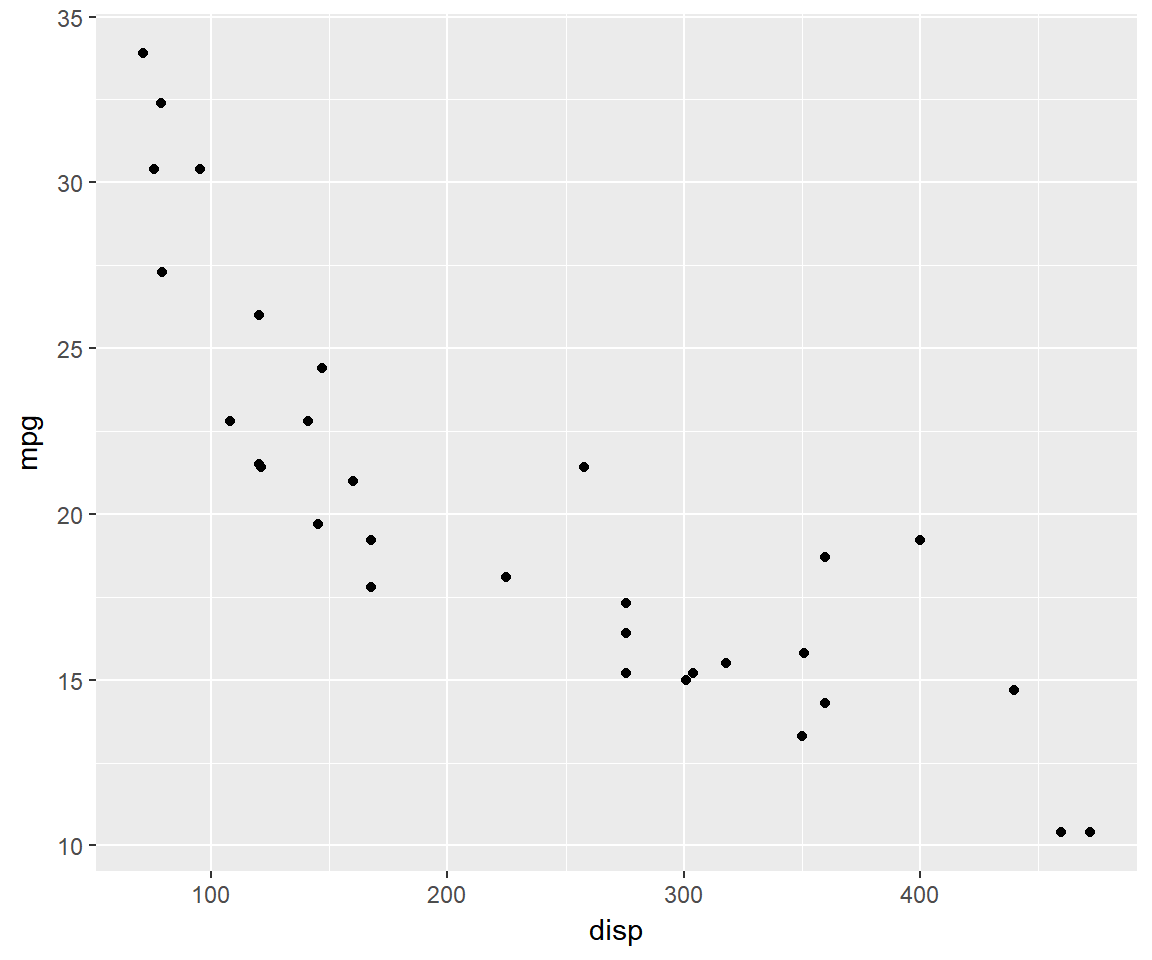## Agenda

• build scatter plots
• modify point
• color
• fill
• alpha
• shape
• size
• fit regression line

## Intro

A scatter plot displays the relationship between two continuous variables. In ggplot2, we can build a scatter plot using `geom_point()`. Scatterplots can show you visually

• the strength of the relationship between the variables
• the direction of the relationship between the variables
• and whether outliers exist

## Libraries

``````library(ggplot2)
library(dplyr)
library(tidyr)``````

## Basic Plot

``````ggplot(mtcars) +
geom_point(aes(disp, mpg))``````## Jitter

``````ggplot(mtcars) +
geom_point(aes(disp, mpg), position = 'jitter')``````